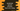# How to remove all zeros from a number string in JavaScript## How to remove all zeros from a number string in JavaScript:

In this post, we will learn how to remove all zeros from a number represented as string in JavaScript. If the string holds only zeros, just return 0. We will learn different ways to solve this problem in this post.

### Method 1: By traversing the characters of the string:

This is the naive approach to solve this problem. Traverse the string characters from left to right. If you find any non-zero character, add all characters from this to end to a new result string.

Let’s learn how to do that with an example program:

``````const removeZeros = (str) => {
if(str === undefined || str === ''){
return str;
}

let i = 0;

while(str[i] === '0' && i < str.length){
i++;
}

return i === str.length ? '0' : str.substr(i);
}

let testStrings = ['0000', '', '0', '0012', '001200', '001200120012', 'hello', '0hello0', undefined];

for(let i in testStrings){
console.log(`\${testStrings[i]} ==> \${removeZeros(testStrings[i])}`);
}``````

Here,

• removeZeros function is used to remove all zeros from a given string. It takes a string as the parameter, removes all leading zeros and returns the new string.
• If the string is an empty string or undefined, it returns the same string.

• Else, it uses a while loop to iterate over the leading zeros.
• If all characters are zero, or if the while loop moves the value of i equal to the string length, it returns 0, else it returns the sub string from i to the end of that string.
• testStrings is an array of strings. The for loop iterates through the strings and prints the results for each string.

If you run this program, it will print:

``````0000 ==> 0
==>
0 ==> 0
0012 ==> 12
001200 ==> 1200
001200120012 ==> 1200120012
hello ==> hello
0hello0 ==> hello0
undefined ==> undefined``````

As you can see that it removes all leading zeros from the strings. If all are zero, it returns 0.

### Method 2: By using replace:

We can also use the replace method. This is an inbuilt method and it can be used to replace all matches by a regular expression by another string. It takes two parameters. The first one is the regular expression to match the characters to replace and the second one is a string to replace the matched values.

If we want to match all the leading zeros of a string, we can pass /^0+/ as the regular expression.

Let’s write down the program:

``````const removeZeros = (str) => {
if(str === undefined){
return str;
}

return str.replace(/^0+/, '');
}

let testStrings = ['0000', '', '0', '0012', '001200', '001200120012', 'hello', '0hello0', undefined];

for(let i in testStrings){
console.log(`\${testStrings[i]} ==> \${removeZeros(testStrings[i])}`);
}``````

That’s only one line. Also, we can remove the check for empty strings from the if statement.

It will print the same result:

``````0000 ==>
==>
0 ==>
0012 ==> 12
001200 ==> 1200
001200120012 ==> 1200120012
hello ==> hello
0hello0 ==> hello0
undefined ==> undefined``````You can also add few more strings to the array to test with different types of strings.

### Method 3: By using parseInt or parseFloat:

If you are sure that the inputs are always integers or floats, you can use these two functions:

• parseInt
• parseFloat

The parseInt function parses a string argument and returns an integer. Similarly, the parseFloat function parses a string argument and returns a float.

Let’s try parseInt with the same set of strings:

``````const removeZeros = (str) => {
return parseInt(str).toString();
}

let testStrings = ['0000', '', '0', '0012', '001200', '001200120012', 'hello', '0hello0', undefined];

for(let i in testStrings){
console.log(`\${testStrings[i]} ==> \${removeZeros(testStrings[i])}`);
}``````

It will print:

``````0000 ==> 0
==> NaN
0 ==> 0
0012 ==> 12
001200 ==> 1200
001200120012 ==> 1200120012
hello ==> NaN
0hello0 ==> 0
undefined ==> NaN``````

parseInt will fail for floating point values. We have to use parseFloat:

``````const removeZeros = (str) => {
return parseFloat(str).toString();
}

let testStrings = ['00.123', '12.344', '9292.12', 'hello', '12.34hello'];

for(let i in testStrings){
console.log(`\${testStrings[i]} ==> \${removeZeros(testStrings[i])}`);
}``````

It will work:

``````00.123 ==> 0
12.344 ==> 12
9292.12 ==> 9292
hello ==> NaN
12.34hello ==> 12``````

But, if you are not sure about the types of the input strings, you should avoid this method.